### Understanding finance is required to sell renewable energy projects. It’s needed to communicate the value of both residential and commercial projects, and for all types of technologies: solar PV, solar hot water, and geothermal heat pumps.

The reason financial metrics are important is that all of these technologies are financial investments.  Thus, you must be able to communicate the financial value of the system to the client and ‘payback period’ does not do this. I repeat, don’t use ‘payback period’, and we’ll talk about why later.

The key to understanding financial analysis is a small contradiction. The actual financial calculations are not difficult once you have all the numbers. The challenging aspect of financial analysis is that many of the numbers the model depends on are assumptions and projections — things you can’t always nail down. Thus, it’s important to perform sensitivity analysis to see how a few critical variables will impact a project’s returns.

Another challenge is communicating exactly what these numbers mean to a consumer, so they understand it. In order to do this, you need to understand what each term represents and how to explain it in plain language.

I’ve noticed that the information and educational resources on basic financial analysis for the renewable energy industry is lacking. While many PV installers can derate conductors easily, they may not know what the NPV of an array is.  Most geothermal contractors can size of a heat pump, but few know that the typical IRR of a system is when it’s replacing an oil boiler. We need to change this.

Below are the basic financial terms you will need to understand to perform financial analysis on any renewable energy project. I’m simply going to discuss what each variable is and how to calculate it, with an example from excel. At the bottom of the article you’ll be able to download the excel file, so you can play with it yourself.

It’s critical to remember that the variables that impact these metrics will change based upon technology and incentives, but the underlying cash flows that create the financial returns will remain the same. NPV is NPV.

Here are the terms will will discuss

1. Net Present Value (NPV)
2. Present Value
3. Future Value
4. Discount Rate
5. Internal Rate of Return (IRR)
6. Sensitivity Analysis

## Net Present Value (NPV)

NPV is the most recognized metric used to analyze capital projects. NPV takes every known cash flow in a period, negative and positive, and discounts back to today to see if the project is profitable or not.  If a profit has a negative NPV, it should not be completed. If it’s zero, it doesn’t matter if a project is completed or not, from a pure financial perspective. If it’s positive, all else equal, it means the project should be completed.

Unlike ‘payback period’, NPV provides a specific dollar amount that you can use to determine if a project is profitable or not. HOWEVER, NPV analysis can vary widely because it is extremely dependent on the discount rate used. On residential sales in particular, an acceptable discount rate can change greatly depending on the customer.

The analysis can also vary widely due to the confidence one has in the financial assumptions used to create the model. It is key to perform a sensitivity analysis when performing NPV analysis because most times the cash values being used are projections and it cannot be said with 100% confidence the numbers will be exact.

The equation to calculate NPV is to add together the present values of each cash flow for each period for a project. Here is the formula to calculate present value for a single period.

Present Value = Net Cash Flow / (1 + i)^t

i = discount rate

t = time period.

**Note, I’m using “^” meaning to the power of X, or in replacement of a supercript because our publishing software does not allow superscript. This is also the same script that excel will use if you want to raise a integer to a power of X**

If we had 5 periods, we could calculate the present value for each period, then add those numbers together.

What is the net present value of \$500 investment, with 5 unequal cash flows, 50, 200, 200, 300, and 300 at a 5% discount rate?

Figure 1: Adding together the present values of 5 future cash flows to determine NPV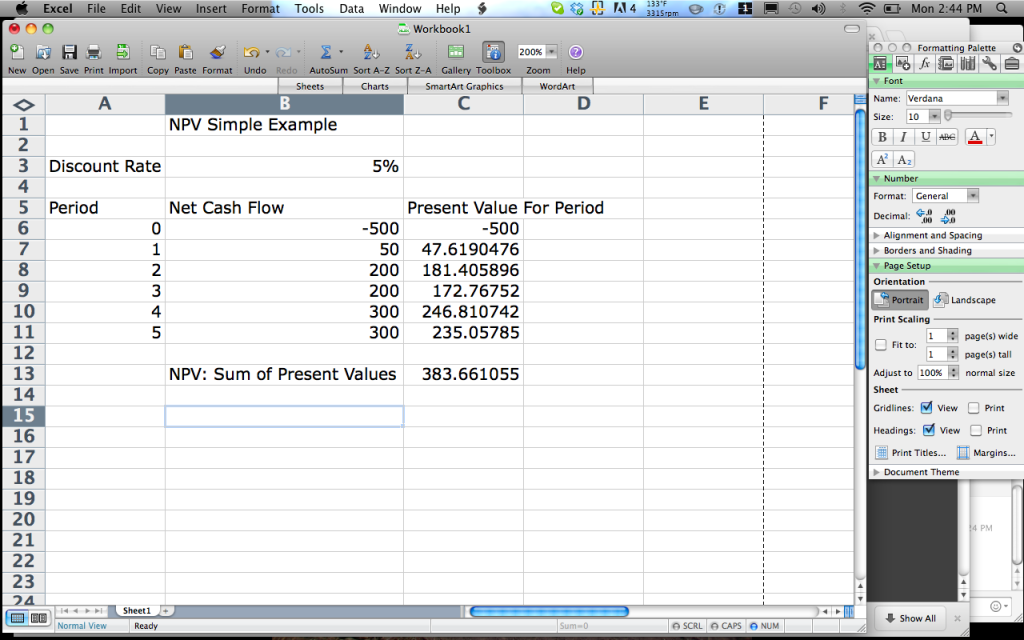A few notes: The cell in C13 is simply summing the values of C6:C11. Each of the values in C6:C11 is calculating the present value of a single cash flow. Notice how \$200 in 2 years, is worth more then \$200 in year 3? This is because it’s getting discounted by 5% every year.

## Present Value (PV):

Present value is the present value, today, of a future cash amount discounted back to today. Net present value is thus, a series of cash flows all discounted back to today’s terms. For example, what is \$50 worth today? It’s worth \$50. However, if you wanted to find out what \$100 in 5 years would be worth today at a 5% interest rate, you’d need to calculate the present value. Here is the equation.

The equation to find present value of a future cash flow is:

PV = FV / (1 + i) ^ n

i = interest rate

n = number of period.

So, what is the present value of \$100 payment in 5 years at a discount rate of 5%

PV = \$100 / (1 + .05) ^ 5

PV = \$100 / 1.28

PV = \$78.15

This means that is someone gave you \$100 in 5 years, and you have a bank account with a yield of 5%, it would have been the same value of money if they would have given you \$78.15 today and you put the money into the bank for 5 years.

## Future Value: (FV)

The future value is asking what the future value is of a present day cash amount, given it is accumulating at a specific interest rate. The best way of describing future value is a typical savings accounts.

If you put \$50 dollars into a savings account with a 5% interest rate and take it out in 10 years, how much will it be worth?

The equation to calculate future value is

FV = PV (1+i)^n.

FV = the value of a future cash flow today, given x % interest rate.

PV = the present value of the investment

i = the interest rate of the investment

n = number of periods of the investment

FV = \$50 (1+.05) ^ 10

(1.05)^10 = 1.63

\$50 * 1.63 = \$81.44

In other words, \$50 today at 5% interest is EQUAL TO be given \$81.44 in 10 years

How about a 10% interest rate?

FV = \$50 (1 + .10) ^10

FV = \$129.69

As you can see, the interest rate used over the term has a huge impact on the value of the investment.

## Discount Rate / Interest Rate:

In the calculations of NPV, PV, and FV, you’ve noticed that we’ve been using an interest rate to calculate the value of money in different parts of time. This value is called the discount rate. Sometimes, it’s referred to as the interest rate (for future value), or minimal attractive rate of return (MARR), which we’ll discuss below.

The discount rate can be somewhat confusing to some. There are critical pieces to understand about the discount rate. First, what it does. Second, how you determine it.

In the above examples of calculating PV and FV you noticed I used an interest rate to calculate the value of cash between a certain  period in time and another period in time. So, to define it very simply the discount rate is an interest rate that is the difference between a present value and future value of the same dollar amount. The difference between \$100 today and in five years is the discount rate.

How one should select the discount rate is a little more difficult. Many times the discount rate is selected based on a few characteristics. None of these is wrong, it simply depends on the circumstances.

1. A comparable investment or savings rate. If a homeowner could invest the same money in a CD at risk free interest rate of 5.6%, they will likely use 5.6% as a discount rate for other investments. Also, keep in mind that many times a homeowner might add a few percentage points to a different investment that is not risk free to cover the additional risk.
2. The inflation rate. If I had \$100 in cash and stuffed it in a safe (a place that is not getting interest), and took it out in 5 years, it would have lower purchasing power. To understand how the purchasing power changes, we would calculate the FV of \$100 in 5 years with the discount rate being the expected rate of inflation.
3. Risk tolerance. The more risky the investment, the higher discount rate you’d need to satisfy the level or risk. Having a higher discount rate will decrease the time it takes for you re-coup your investment, given the NPV is still positive. When risk tolerance is being used to determine need returns, it’s sometimes referred to as “Minimum Attractive Rate of Return” (MARR), or the “hurdle rate”.

The thing to remember about discount rate is that while it’s use in the financial analysis is extremely clear, determining what exactly to use as a discount rate is extremely subjective or will vary widely between homeowners.

The impact of a different discount rate can be huge when talking about renewable energy projects because an acceptable discount rate between different homeowners can vary widely. Let’s walk through some examples to demonstrate.

CASE STUDY: A Sample Solar Hot Water Customer in Greenfield, MA.

• Net Installed Cost After Incentives: \$4,000
• Displaced Oil : 130 Gallons with 3 Full Time Occupants
• Value of Displaced Oil @ 3.00 Gallon = \$390
• The life of the system will be 20 years.
• Maintenance costs are \$200 at year 10.
• All other equipment failures will be paid by the manufacturer
Here’s the T*Sol estimation for the system production and load.

## Sally the Solar Hot Water Customer

Let’s say that Sally has a discount rate of 16%. Why? Sally has an outstanding credit card debt of \$4,000. Thus, the investment in a SHW system must make money then her credit card payments or she might as well pay of the credit card first.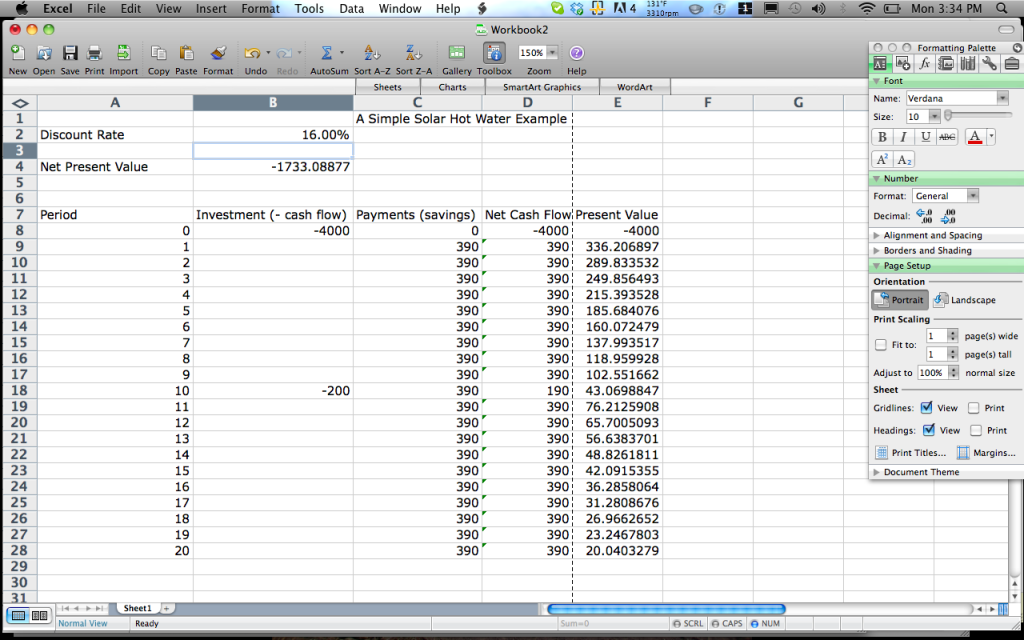Notice that at a 16% discount rate, the net present value of the investment is worth        -\$1,733. The reason for this is simple, when the interest rate is so high the money quickly looses it’s value. In 9 years, at 16% a \$360 dollar savings in today’s dollars is only worth \$102.

## Now, let’s say we have a customer named Ralph.

Ralph doesn’t have any credit card debt and he has an extra \$4,000 on his home equity line of credit. His line of credit has an interest rate of 4.5%. Thus, his discount rate is 4.5% because that is the INTEREST payment he will need to pay. Thus, he needs to make MORE THAN his interest payment for the SHW system to be profitable.As you can see, Ralph’s net present value is a positive \$944 dollars over the twenty year period. This means if Ralph borrows \$4,000 a 4.5% FIXED interest rate his will save \$944 in today’s dollars over the next 20 years. Basically, it’s free money.

Energy Inflation Rates: Now, I know what a bunch of you are saying, “Chris oil WILL NOT stay at \$3.50 a gallon for the next 20 years!” Well, I absolutely agree with you and we’ll discuss this in the sensitivity analysis section.

## Internal Rate of Return (IRR):

The internal rate of return is the discount rate that will make a series of nominal cash flows (not discounted to present value) have a NPV of ZERO. When the NPV of an investment is ZERO, it means that making the investment or not making the investment will provide the same return. Thus, many times an investment’s IRR is compared to the actual cost of capital or comparison investment. A good way of thinking about the definition is that the IRR is a special kind of discount rate that makes it easy to compare the impact of various discounts rates on the same investment.

Let’s continue the same example from above with A Very Simple SHW Project. In order to calculate the IRR, we use the “=IRR” function in excel and include all the nominal cash flows from the project.When we hit select it calculates the IRR at 9%The IRR has nothing, directly, to do with the calculattion of the NPV or the discount rate. However the cash flows that help to determine IRR, are what we used to calculate NPV.

Notice, how the discount rate in our example from Ralph is 4.5% and our NPV is \$1,444. If we change the discount rate to match the IRR, the NPV will get close to zero, not exactly due to rounding.

The IRR is the same, 9%, because it is determined based on the nominal cash flows. The discount rate and NPV are also connected because you need a discount rate to calculate the NPV.IRR is extremely useful when dealing with homeowners for a few reasons. First, it provides a very clear metric of understanding the investment. Because homeowners have so many different hurdles rates, providing them a clear number is very useful.

So, in our example the IRR is 9%, given the cash flows.

Thus, when comparing Sally and Ralph, the decision is clear.

Sally’s Minimal Acceptable Return is 16%, due to other debts, so it does not make sense for her to invest in the solar project because 16% is more then 9%.

However, because Ralph does not have any debt and he can acquire cash for 4.5%, he just needs to make more money then 4.5%. Because 9% is higher then 4.5%, Ralph is going to make money on this deal.

# Sensitivity Analysis:

Sensitivity analysis is the critical element in financial analysis for renewable energy projects. Why? Because calculating NPV and IRR is very simple. However, the difficulty is assigning a level of confidence to the variables that you are using in your analysis. Most all of the variables are projections so the question becomes what numbers are more concrete then others and what happens if some key numbers are a little, or a lot, higher or lower then expected.

To create a proper sensitivity analysis you need to understand the variables of a renewable energy installation that will impact the installation and how they might change.

Here are they key variables about most renewable energy projects you’ll need to understand. Right now, I’m going to speak about them in general and will address technology specific applications of each, in the technology specific posts.

1. The net initial cost of the system. Gross installed system cost minus grants or rebates.

Calculating this number can get get tricky with commercial solar PV projects due to when MACRS is applied and the value it has. However, for this post, we’re going to stick to our sample problem.

What happens to the IRR of the project when the net installation costs goes from \$4000 to \$5000?It decreases from 7.10% to 4.37%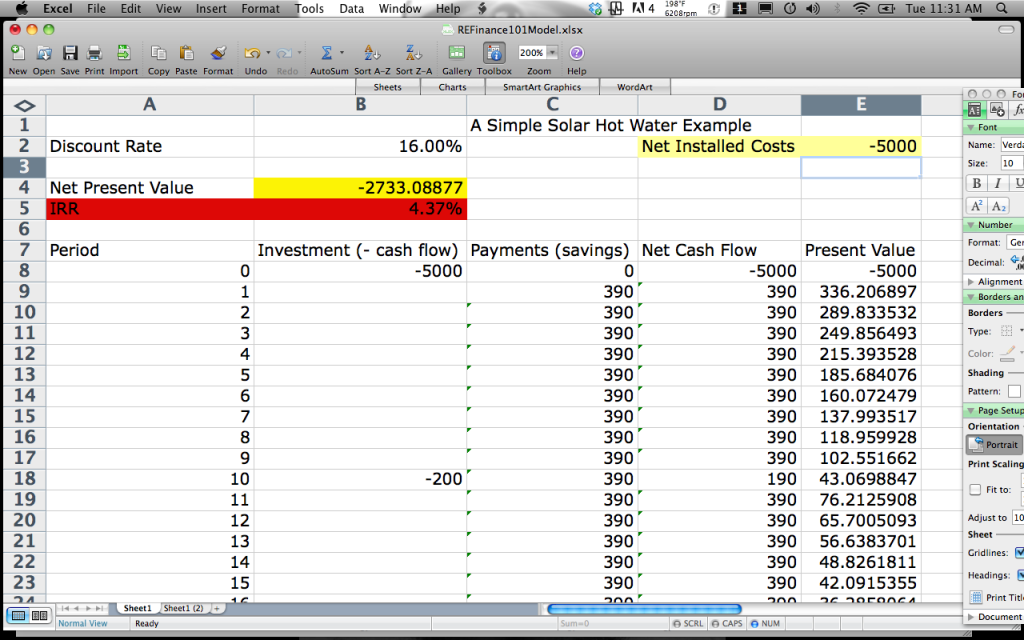2. Maintence cost of the systems.

The maintenance costs of the system are show in column B as investments or an expense and impact the net cash flow for a single period.

Large maintenance costs do have the ability to impact returns and it’s why it’s very important to be clear with the homeowner about what is warrantied under a manufacturers equipment warranty, installation warranty and what they will need to pay themselves.

The system lifetime actually has a huge impact on the expected IRR’s of a project.

It’s also hotly debated what the lifetime of a system is. Many times equipment will have a warranty of 20 years and the system lifetime will be calculated at 20 years, even though its very likely it will be running longer then 20 years. But the question remains, what will the maintenance costs be after 20 years? It’s impossible to predict.

Also, less information is known the further you move away from the present, so many just feel comfortable for 20 years.

To show an example, if you take our sample and reduce the life of the system from 20 years to 15 years, the IRR drops significantly from 7.10% to 4.2%.

20 year life to 15 year life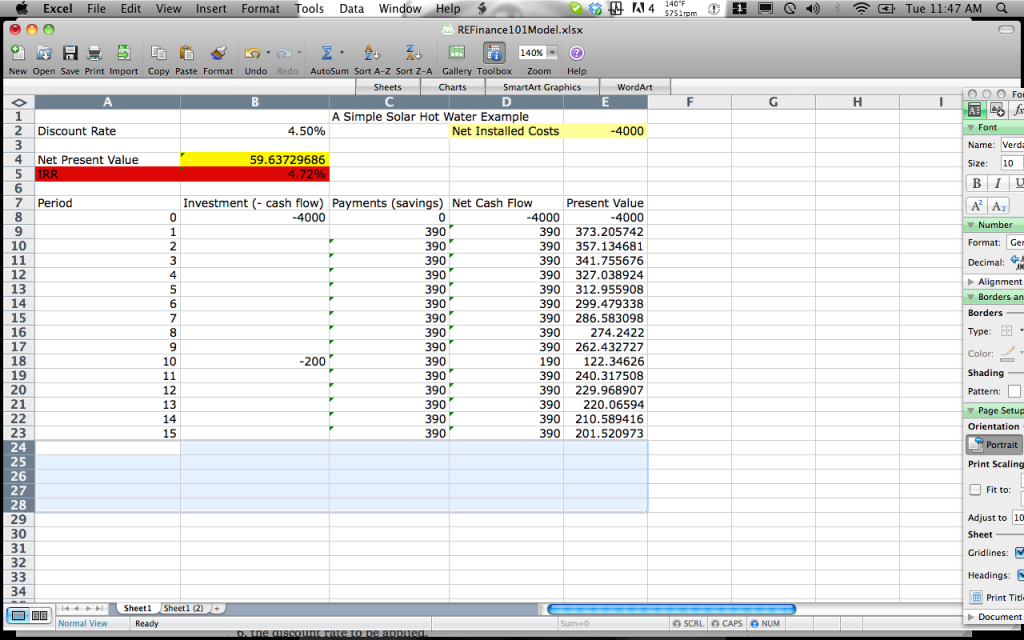4. The amount of energy created and the value of that energy, including production based incentives like SRECS.

For solar thermal systems, we’re creating BTUs. However, the VALUE of the BTUs that they are offsetting will change in almost every building and over time. Why? The cost of a BTU is determined by three variables

1. Cost of the fuel source
2. Efficiency of the heating element
3. Inflation rate of the energy source.

For simplicity purposes, let’s assume the software that we use takes into consideration the efficiency of the heating element and simply provides us with the quantity of fuel our SHW system will displace. In our example, that’s 130 gallons of #2 heating oil per year.

What happens to our numbers if the average cost of oil will actually be higher then this? What happens if the average oil inflation rate over 20 years is 5% versus 2%?

I started the example with oil costs a \$3.00 per gallon to be conservative, currently one March 20th 2012, they’re around \$4.00, what happens to the return of oil stay at \$3.00 per gallon or \$4.00 for the next 20 years?Oil @ \$4.00 gallon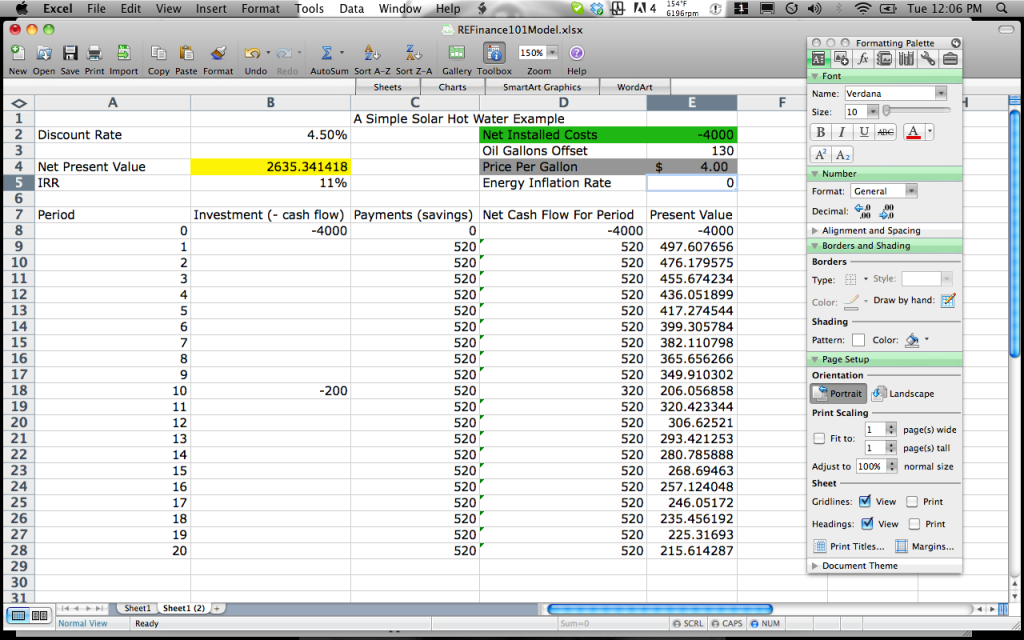Notice that a 33% increase in energy costs, oil from \$3 to \$4, the IRR of the project increased by 57%, from just over 7% to just over 11%. The NPV of the project increased by 180% from \$944 to \$2,635.

Now lets look at the impact of energy inflation. Let’s assume oil will start at \$3.50 a gallon today, to be conservative, and lets see the difference between an inflation rate of 2% (conservative) to 6% (optimistic, from a SHW perspective).

0% Inflation Rate at \$3.50 for the next 20 years

• IRR = 9.24%
• NPV = \$1790

2% Oil Inflation Rate Starting a \$3.50 a Gallon

• IRR = 11.19%
• NPV = \$2857

6% Oil Inflation Rate Starting at \$3.50 a gallon

• IRR = 15.06%
• NPV = \$5875

As you can see, this is why sensitivity analysis is critical, the financial returns are much better with higher inflation. However, there is no way of determining with any certainty what the cost of oil will be in 20 years. That’s why it always makes sense to be as conservative as possible.

5. The discount rate to be applied.

The discount rate is the factor that will have the largest impact on the value of the investment and is also the hardest to determine for each homeowner. We saw the example with Sally and Ralph in a simple example.

When applying a discount rate, just make sure that it is based on some, logical, evidence and assumptions. If you cannot find anything, between 4% and 5% is standard.

## Other considerations not taken into financial analysis that property owners care about.

The major consideration not taken into account for financial analysis that  has a huge value in consumers minds is the appearance, symbolism and the clients politics around why they are interested in solar. Many times, these “soft benefits” might be more important to a consumer then the actual NPV of the system.

While doing the site visit, make sure to qualify the customer properly so you know exactly what they’re getting solar. Use their interests in solar to custom tailor each sales proposal.

## Payback: Why I won’t Use it.

Many people use the term payback, I do not, and think it’s a useless term. Since the future savings generated by a renewable energy project are unlikely to be constant and because it ignores the time value of money, payback is not suitable for this analysis.

Payback is best suited for high risk investments, not well known technologies that have a 20 year life.